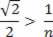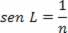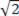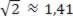# prisms

In prisms, there are two refractions with angular deviation, one on each face.

In the figure above we see a block of glass, in the shape of a triangular prism. The light, after falling on the left face of the prism, undergoes two refractions. In optics, we define a prism as a set of three homogeneous and transparent media (air, glass, air, for example), separated by two flat and non-parallel surfaces.

total reflection prism

It is common, in certain prisms, for the light ray to undergo refraction on the first face and total reflection on the second face. In this case, the prism is called a total reflection prism.

The figures below present the two most commonly used total reflection prisms in practice. Both are shaped like a right triangle and isosceles, but they are in different positions in relation to the incident light.

In the Amici prism, the emerging ray is perpendicular to the incident ray, due to total reflection. In the Porro prism, the emerging ray has the same direction as the incident ray, but in the opposite direction. Total reflection prisms have great practical application, mainly as a replacement for plane mirrors in optical instruments.Resolution:The angle at which the ray strikes the second face of the prism must be greater than the threshold angle. Thus, 45º > L, therefore, sin 45º > sin L. Then:

Because:

So n >. Remembering that, we have: n > 1.41

Check Also
Close